• +91 9971497814
• info@interviewmaterial.com

# Chapter 2- Structure of The Atom Interview Questions Answers

### Related Subjects

Question 1 : (i) Calculate the number of electrons whichwill together weigh one gram.
(ii) Calculate the mass and charge ofone mole of electrons.

Answer 1 : (i) Mass of an electron = 9.1 × 10-28 g
9.1 × 10-28 g is the mass of = 1 electron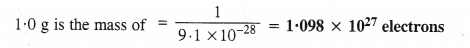(ii) One mole ofelectrons = 6.022 × 1023 electrons
Mass of 1 electron = 9.1 × 10-31 kg
Mass of 6.022 × 1023 electrons = (9.1 × 10.31kg) × (6.022 × 1023)= 5.48 × 10-7 kg
Charge on one electron = 1.602 × 10-19 coulomb
Charge on one mole electrons = 1.602 × 10-19 × 6.022 × 1023 =9.65 × 104 coulombs

Question 2 : (i) Calculate the total number of electronspresent in one mole of methane.
(ii) Find (a) the total number and (b)the total mass of neutrons in 7 mg of 14C. (Assume that mass ofa neutron = 1.675 × 10-27kg).
(iii) Find (a) the total number and (b)the total mass of protons in 34 mg of NH3 at STP.
Will the answer change if thetemperature and pressure are changed ?

(i) One mole ofmethane (CH4) has molecules = 6.022 × 1023
No. of electrons present in one molecule of CH4 = 6 + 4 = 10
No. of electrons present in 6.022 × 1023 molecules of CH4 =6.022 × 1023 × 10
= 6.022 × 1024 electrons

(ii) Step I. Calculation of totalnumber of carbon atoms
Gram atomic mass of carbon (C-14) = 14 g = 14 × 103 mg
14 × 103 mg of carbon (C-14) have atoms = 6.022 × 1023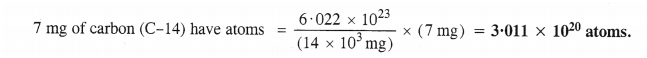Step II. Calculation oftotal number and tatal mass of neutrons
No. of neutrons present in one atom (C-14) of carbon = 14 – 6 = 8
No. of neutrons present in 3-011 × 1020 atoms (C-14) of carbon= 3.011 × 1020 × 8
= 2.408 × 1021 neutrons
Mass of one neutron = 1.675 × 10-27 kg
Mass of 2.408 × 1021 neutrons = (1.675X10-27 kg)× 2.408 × 1021
= 4.033 × 10-6 kg.

(iii)Step I. Calculation of totalnumber ofNH3 molecules
Gram molecular mass of ammonia (NH3) = 17 g = 17 × 103 mg
17 × 103 mg of NH3 have molecules = 6.022 × 1023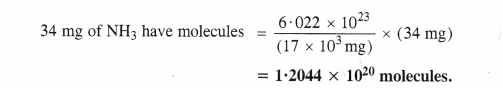Step II. Calculation oftotal number and mass of protons
No. of protons present in one molecule of NH3 = 7 + 3 = 10 .
No. of protons present in 12.044 × 1020 molecules of NH3 =12.044 × 1020 × 10
= 1.2044 × 1022 protons
Mass of one proton = 1.67 × 10-27 kg
Mass of 1.2044 × 1022 protons = (1.67 × 10-27 kg)× 1.2044 × 1022
= 2.01 × 10-5 kg.
No, the answer will not change upon changing the temperature and pressurebecause only the number of protons and mass of protons are involved.

Question 3 : How many protons and neutrons are present inthe following nuclei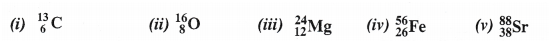Answer 3 :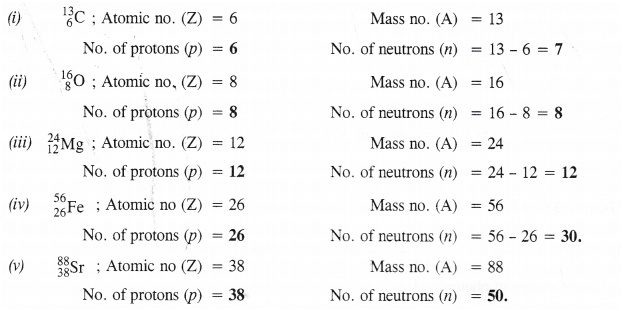Question 4 : Write the complete symbol for the atom (X)with the given atomic number (Z) and atomic mass (A)
(i) Z = 17,A = 35
(ii) Z = 92, A = 233
(in) Z = 4, A = 9.

Answer 4 :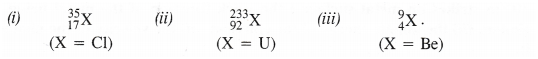Question 5 : Yellow light emitted from a sodium lamp has awavelength (2) of 580 nm. Calculate the frequency (v) and wave number (v) ofyellow light.

Answer 5 :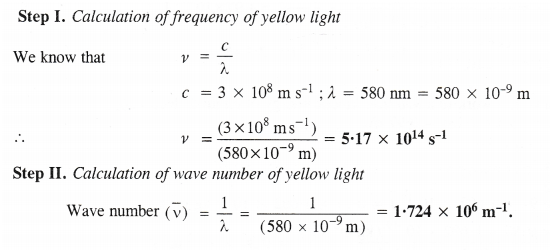Question 6 : Calculate the energy of each of the photonswhich
(i) correspond to light of frequency 3× 1015 Hz
(ii) have wavelength of 0-50 A.

Answer 6 : (i) Energy of photon (E) = hv
h = 6.626 × 10-34 J s ; v = 3 × 1015 Hz = 3 ×1015s-1
E = (6.626 × 10-34 Js) × (3 × 1015 s-1) = 1.986 × 1018 J
Energy of photon (E) = hv = hcλ
h = 6.626 × 10 34 J s; c = 3 × 108 m s-1 ;
λ= 0.50 Å = 0.5 × 10-10 m.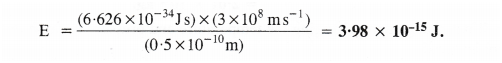Question 7 : Calculate the wavelength, frequency, andwavenumber of lightwave whose period is 2.0 × 10-10 s.